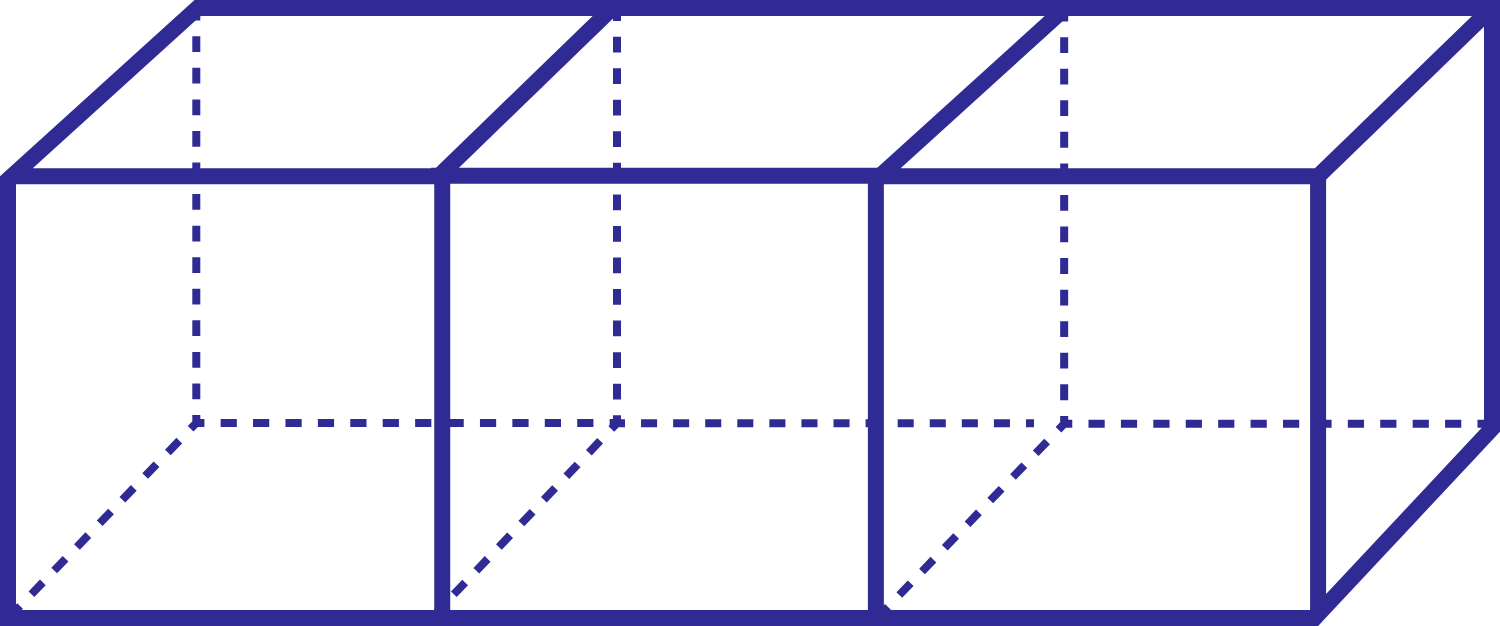Cubes form Cuboids

Geometry Level 1

Three cubes of side length 1 are joined together (side by side) to form a cuboid, what is the ratio of the surface areas of one of the cubes to the cuboid?

The answer is of the form $\dfrac ab$, where $a$ and $b$ are coprime positive integers.

Submit the answer as $a+b$.×